Foxtable(狐表)用户栏目专家坐堂 → [求助]求遍历字符组的计数如何做，分数，几分之几

共有163人关注过本帖树形打印复制链接

# 主题：[求助]求遍历字符组的计数如何做，分数，几分之几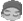myjht
1楼 | 信息 | 搜索 | 邮箱 | 主页 | UC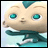加好友发短信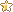[求助]求遍历字符组的计数如何做，分数，几分之几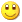Post By：2021/4/8 10:59:00 [只看该作者]

 求如下图，规格列“3左+3右”，按“+”拆分后计数，和当前打印第几个的语句如何写，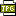此主题相关图片如下：微信图片_20210315102558.jpgDim doc As New PrintDoc '定义一个报表'添加打印内容Dim img As prt.RenderImage '定义一个图片对象Dim Bar As New BarCodeBuilderBar.Symbology = Barpro.Symbology.Code39'Bar.Rotate = Barpro.Rotate.Degree90 '旋转条码For Each r2 As Row In Tables("zzscjjzb").Rows    Dim nms() As String = r2("规格").Split("+") '将规格列内容拆分成数组    For k As Integer = 1 To r2("数量") '加一个循环        For Each nm As String In nms '遍历参与加工此产品的每个工号                        '下面很简单,指定每一个单元格的内容            rt.Cells(0,0).Text= "工单号"            rt.Cells(0,1).Text = r2("工单号")            rt.Cells(4,0).Text= "产品名称"            rt.Cells(4,1).Text = r2("产品名称")            rt.Cells(5,0).Text= "颜色"            rt.Cells(5,1).Text = r2("颜色")            rt.Cells(6,0).Text= "件数"            rt.Cells(6,1).Text = r2("件数")            rt.Cells(7,0).Text= "数量"            rt.Cells(7,1).Text = r2("数量")                        If r2("规格") = "" Then                rt.Cells(8,0).Text= "套规格"                rt.Cells(8,1).Text = nm            Else                rt.Cells(8,0).Text= "套规格"                rt.Cells(8,1).Text = r2("规格") & "  /  " & nm            End If                        rt.Cells(9,0).Text= "单据备注"            rt.Cells(9,1).Text = r2("单据备注")            rt.Cells(10,0).Text = r2("非标说明")                        bar.Code = r2("_Identify")            img = new prt.RenderImage            img.Image = bar.GetImage            img.Height = 13 '"Auto" '高度由图片大小自动决定                        ra.Children.Add(rt) '加入到容器中            ra.Children.Add(img) '加入图片到RenderArea中                        Doc.Body.ChildRen.Add(ra) '将容器加入到报表中                    Next    NextNextDoc.Preview() '预览报表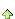myjht
2楼 | 信息 | 搜索 | 邮箱 | 主页 | UC加好友发短信Post By：2021/4/8 11:18:00 [只看该作者]

 下载信息  [文件大小：   下载次数： ]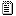点击浏览该文件:代码.txt3楼 | 信息 | 搜索 | 邮箱 | 主页 | UC加好友发短信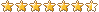Post By：2021/4/8 11:20:00 [只看该作者]

 这个意思？Dim nms() As String = r2("规格").Split("+")For i as integer = 0 to nms.length - 1msgbox((i+1) & "/" & nms.length)nextmyjht
4楼 | 信息 | 搜索 | 邮箱 | 主页 | UC加好友发短信[求助]求遍历字符组的计数如何做，分数，几分之几Post By：2021/4/8 14:56:00 [只看该作者]

 感谢篮版，不过我加到那个位置合适？我的循环冲突了Dim doc As New PrintDoc '定义一个报表For Each r2 As Row In Tables("zzscjjzb").Rows    Dim nms() As String = r2("规格").Split("+") '将规格列内容拆分成数组    For k As Integer = 1 To r2("数量") '加一个循环                For i As Integer = 0 To nms.length - 1                        For Each nm As String In nms '遍历参与加工此产品的每个工号                rt.Cells(4,1).Text = r2("产品名称")                rt.Cells(5,0).Text= "颜色"                rt.Cells(5,1).Text = r2("颜色")                rt.Cells(6,0).Text= "件数"                rt.Cells(6,1).Text = r2("件数")                rt.Cells(7,0).Text= "数量"                rt.Cells(7,1).Text = r2("数量")                                If r2("规格") = "" Then                                        rt.Cells(8,0).Text= "套规格"                    rt.Cells(8,1).Text = nm                Else                    rt.Cells(8,0).Text= "套规格"                    'rt.Cells(8,1).Text = r2("规格") & "  /  " & nm                                        rt.Cells(8,1).Text = r2("规格") & "  /  " & nm  &  (i+1) & "/" & nms.length                                    End If                ra.Children.Add(rt) '加入到容器中                ra.Children.Add(img) '加入图片到RenderArea中                                Doc.Body.ChildRen.Add(ra) '将容器加入到报表中            Next        Next    NextNextDoc.Preview() '预览报表5楼 | 信息 | 搜索 | 邮箱 | 主页 | UC加好友发短信Post By：2021/4/8 15:22:00 [只看该作者]

 For k As Integer = 1 To r2("数量") '加一个循环                For i As Integer = 0 To nms.length - 1                        '删除这行For Each nm As String In nms '遍历参与加工此产品的每个工号dim nm as string = nms(i)                rt.Cells(4,1).Text = r2("产品名称")                rt.Cells(5,0).Text= "颜色"                rt.Cells(5,1).Text = r2("颜色")myjht
6楼 | 信息 | 搜索 | 邮箱 | 主页 | UC加好友发短信Post By：2021/4/8 16:50:00 [只看该作者]

 感谢篮版，新手小白，憋了好几天，最后一个问题，如果r2（“产品名称”）包含“外阜”这个文字，条码打印4份，否则打印1份，对应的rt.Cells(8,1).Text = r2("规格") & "  /  " & nm  &  (i+1) & "/" & nms.length，也要同步，这个应该怎么写。7楼 | 信息 | 搜索 | 邮箱 | 主页 | UC加好友发短信Post By：2021/4/8 17:01:00 [只看该作者]

 没看懂，意思是总共要打印4 X nms.length 这么多份？myjht
8楼 | 信息 | 搜索 | 邮箱 | 主页 | UC加好友发短信[求助]求遍历字符组的计数如何做，分数，几分之几Post By：2021/4/9 9:37:00 [只看该作者]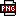此主题相关图片如下：6a723f2e-12b1-49f1-a173-53e7be6d1a62.png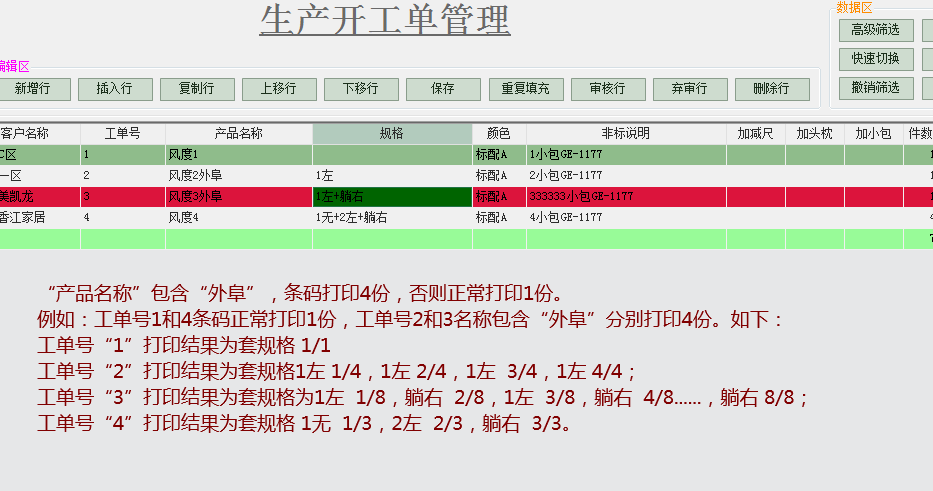下载信息  [文件大小：   下载次数： ]点击浏览该文件:代码.txt9楼 | 信息 | 搜索 | 邮箱 | 主页 | UC加好友发短信Post By：2021/4/9 9:57:00 [只看该作者]

 For Each r2 As Row In Tables("zzscjjzb").Rows    Dim nms() As String = r2("规格").Split("+") '将规格列内容拆分成数组    For k As Integer = 1 To r2("数量") '加一个循环dim k as integer = 0if r2（“产品名称”）like“*外阜*”then k = 3dim g as integer = nms.lengthif k>0 then g = g * (k+1)dim idx as integer = 1for m as integer = 0 to k        For i As Integer = 0 To nms.length - 1            Dim nm As String = nms(i)                        Dim rt As New prt.RenderTable() '定义一个表格对象            Dim rx As New prt.RenderText '定义一个文本对象……                        If r2("规格") = "" Then                                rt.Cells(8,0).Text= "套规格"                rt.Cells(8,1).Text = nm            Else                rt.Cells(8,0).Text= "套规格"                rt.Cells(8,1).Text = r2("规格") & "  /  " & nm &    idx & "/" & gidx += 1            End Ifmyjht
10楼 | 信息 | 搜索 | 邮箱 | 主页 | UC加好友发短信[求助]求遍历字符组的计数如何做，分数，几分之几Post By：2021/4/10 16:48:00 [只看该作者]

 下载信息  [文件大小：   下载次数： ]点击浏览该文件:代码.txt总数 11 1 2 下一页